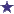# Amoxil 50ml 125ml/5ml Suspension-What is the days supply?

125mg/5ml dispense 50ml
tsp bid

### Comments for Amoxil 50ml 125ml/5ml Suspension-What is the days supply?

Average RatingMar 02, 2022 Rating50ml Amoxil 125/5ml will last 5 days with these directions by: David 1 tsp = 5ml 5ml bid (twice daily) 5ml x 2 (twice daily) = 10ml given daily 10ml given daily x 5 days = 50ml 50ml is the dispensed amount. Another way to figure it is: 50ml divided by 5ml (for each dose) = 10 10 divided by 2 (two doses each day) = 5 days supply.